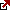# Total Return Formula

Total stock return for stock investors

Most investors usually have multiple options for investing their money. While the investors would like to maximize their investment return, they would also like to ensure that their investment is safe; they can get the principal amount invested back whenever they wish. For many investments like stocks or mutual funds, the investment’s return consists of both the dividend, which the company declares and its value increase. Hence the Total Stock Return, abbreviated as TSR, is the relevant parameter for evaluating stock market investments.

Total stock return definition

The Total stock return or combined stock return includes the total return on the investor’s stock investment. Typically the company will declare a dividend for the stock annually, and the value of the stock could increase, though it may also decrease. For calculating the TSR, the dividend which is declared will be reinvested in the stock. In some cases, the company may also declare some bonus shares for the shareholders of the company. The TSR is usually expressed as a percentage of initial investment, though the cash TSR is calculated in some cases.

The total return on investment formula?

The total return formula represents an equation where the investor divides the total investment gains by the initial value of the investment and multiplies by 100 to convert it to a percentage. For example, if the investor has 100 shares and earning \$15 per share, his investment gains are 100 x \$15 = \$1500.Let the investment’s initial value is \$1000; then the total gain is \$1500-\$1000=\$500. When an investor divides the total investment gains by the initial investment value and multiplies by 100, he will have  \$500/\$1000 x 100 = 20%. Based on the total return on investment formula, the investor’s total return is 20%.

Example numbers:

Initial value: \$1000

Gain: 100 x \$15 = \$1500

Total gains = \$1500-\$1000 = \$500

Total return = \$500/\$1000 x 100 = 20%

## The formula for total return ratio

The formula for total return ratio is:

TSR = ( (P1-P0)+ D )/ P0

where P1 is the value of the stock at the end of the period for which the TSR is being calculated,
P0 is the stock value at the time of purchase,
and D is the dividend declared if any.

The first part is only looking at the increase in the stock price, while the second part is the declared dividend. While most investors prefer to calculate the TSR percentages for the various stocks before finalizing their investment, some investors may wish to use the cash TSR, which is defined as cash TSR = (P1-P0)+ D.

There is also an alternative way of calculating the TSR, combining the increase in stock value and a dividend yield.

TSR = Capital gains yield + Dividend yield for the stock

Importance

One of the reasons why TSR is important for an investor is that various stocks’ performance varies depending on the industry sector. For many of the high dividend yield stocks, the share prices will not increase significantly over a period of time due to low growth potential. In other cases, for a high growth stock, no dividends may be declared, though the stock prices may increase faster. Hence the investor should look at the overall picture, consider both the dividends received and the increase in the stock prices for calculating the TSR.

Typically the investor should check the TSR of the company over a period of several years to find out trends and also compare the TSR with other companies in the same sector. He can also compare the TSR with the benchmark indices for the related sector. This information will help the investor plan his finances and know what returns he can expect from the stock to invest in it. There may be some brokerage and other charges for purchasing the stocks, which are usually not considered for calculations.

Latest posts by Fxigor (see all)

Fxigor

Igor has been a trader since 2007. Currently, Igor works for several prop trading companies. He is an expert in financial niche, long-term trading, and weekly technical levels. The primary field of Igor's research is the application of machine learning in algorithmic trading. Education: Computer Engineering and Ph.D. in machine learning. Igor regularly publishes trading-related videos on the Fxigor Youtube channel. To contact Igor write on: igor@forex.in.rs

Trade gold and silver. Visit the broker's page and start trading high liquidity spot metals - the most traded instruments in the world.Diversify your savings with a gold IRA.

Gold & silver portfolio-building assistance from product professionals.

If you want to trade stocks try: